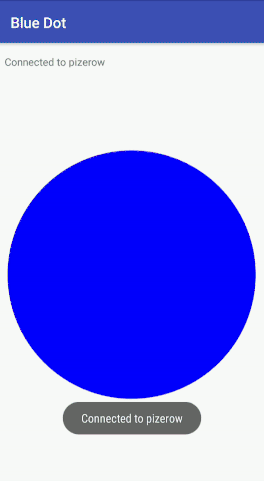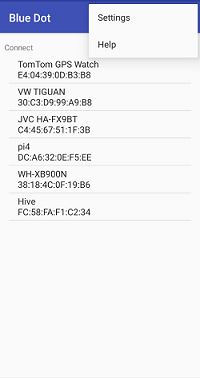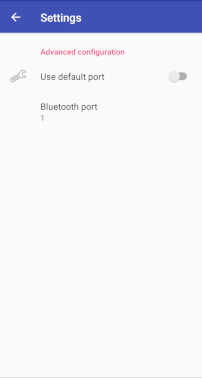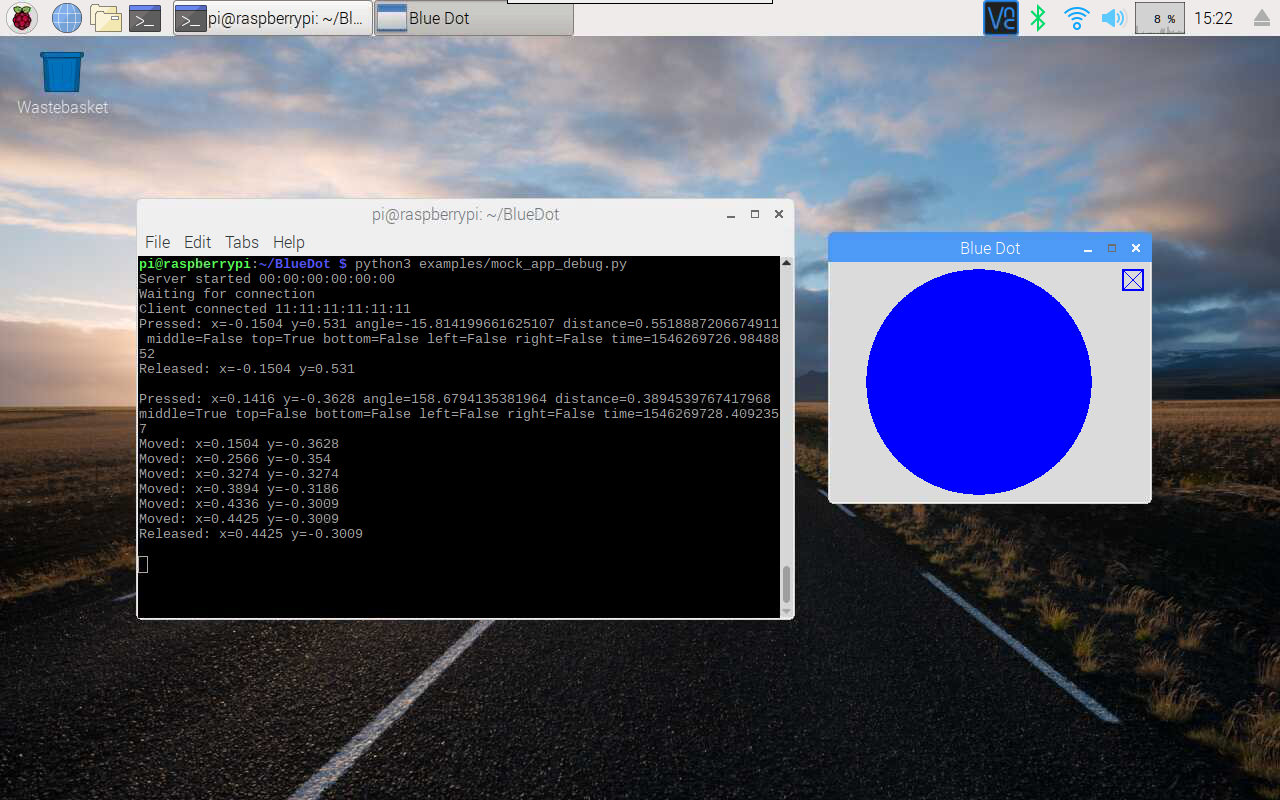# Recipes¶

The recipes provide examples of how you can use Blue Dot. Don’t be restricted by these ideas and be sure to have a look at the Blue Dot API as there is more to be discovered.

## Button¶

The simplest way to use the Blue Dot is as a wireless button.

### Hello World¶

Let’s say “Hello World” by creating the `BlueDot` object then waiting for the Blue Dot app to connect and be pressed:

```from bluedot import BlueDot
bd = BlueDot()
bd.wait_for_press()
print("Hello World")
```

Alternatively you can also use `when_pressed` to call a function:

```from bluedot import BlueDot
from signal import pause

def say_hello():
print("Hello World")

bd = BlueDot()
bd.when_pressed = say_hello

pause()
```

`wait_for_release` and `when_released` also allow you to interact when the Blue Dot is released:

```from bluedot import BlueDot
from signal import pause

def say_hello():
print("Hello World")

def say_goodbye():
print("goodbye")

bd = BlueDot()
bd.when_pressed = say_hello
bd.when_released = say_goodbye

pause()
```

Double presses can also be used with `wait_for_double_press` and `when_double_pressed`:

```from bluedot import BlueDot
from signal import pause

def shout_hello():
print("HELLO")

bd = BlueDot()
bd.when_double_pressed = shout_hello

pause()
```

### Flash an LED¶

Using Blue Dot in combination with `gpiozero` you can interact with electronic components, such as LEDs, connected to your Raspberry Pi.

When the Blue Dot is pressed, the LED connected to GPIO 27 will turn on; when released it will turn off:

```import os
from bluedot import BlueDot
from gpiozero import LED

bd = BlueDot()
led = LED(27)

bd.wait_for_press()
led.on()

bd.wait_for_release()
led.off()
```

You could also use `when_pressed` and `when_released`:

```from bluedot import BlueDot
from gpiozero import LED
from signal import pause

bd = BlueDot()
led = LED(27)

bd.when_pressed = led.on
bd.when_released = led.off

pause()
```

Alternatively use `source` and `values`:

```from bluedot import BlueDot
from gpiozero import LED
from signal import pause

bd = BlueDot()
led = LED(27)

led.source = bd.values

pause()
```

### Remote Camera¶

Using a Raspberry Pi camera module, `picamera.PiCamera` and `BlueDot`, you can really easily create a remote camera:

```from bluedot import BlueDot
from picamera import PiCamera
from signal import pause

bd = BlueDot()
cam = PiCamera()

def take_picture():
cam.capture("pic.jpg")

bd.when_pressed = take_picture

pause()
```

## Joystick¶

The Blue Dot can also be used as a joystick when the middle, top, bottom, left or right areas of the dot are touched.

Using the position the Blue Dot was pressed you can work out whether it was pressed to go up, down, left, right like the D-pad on a joystick:

```from bluedot import BlueDot
from signal import pause

if pos.top:
print("up")
elif pos.bottom:
print("down")
elif pos.left:
print("left")
elif pos.right:
print("right")
elif pos.middle:
print("fire")

bd = BlueDot()

pause()
```

At the moment the D-pad only registers when it is pressed. To get it work when the position is moved you should add the following line above `pause()`:

```bd.when_moved = dpad
```

### Robot¶

These recipes assume your robot is constructed with a pair of H-bridges. The forward and backward pins for the H-bridge of the left wheel are 17 and 18 respectively, and the forward and backward pins for H-bridge of the right wheel are 22 and 23 respectively.

Using the Blue Dot and `gpiozero.Robot`, you can create a bluetooth controlled robot which moves when the dot is pressed and stops when it is released:

```from bluedot import BlueDot
from gpiozero import Robot
from signal import pause

bd = BlueDot()
robot = Robot(left=(17, 18), right=(22, 23))

def move(pos):
if pos.top:
robot.forward()
elif pos.bottom:
robot.backward()
elif pos.left:
robot.left()
elif pos.right:
robot.right()

def stop():
robot.stop()

bd.when_pressed = move
bd.when_moved = move
bd.when_released = stop

pause()
```

### Variable Speed Robot¶

You can change the robot to use variable speeds, so the further towards the edge you press the Blue Dot, the faster the robot will go.

The `distance` attribute returns how far from the centre the Blue Dot was pressed, which can be passed to the robot’s functions to change its speed:

```from bluedot import BlueDot
from gpiozero import Robot
from signal import pause

bd = BlueDot()
robot = Robot(left=(lfpin, lbpin), right=(rfpin, rbpin))

def move(pos):
if pos.top:
robot.forward(pos.distance)
elif pos.bottom:
robot.backward(pos.distance)
elif pos.left:
robot.left(pos.distance)
elif pos.right:
robot.right(pos.distance)

def stop():
robot.stop()

bd.when_pressed = move
bd.when_moved = move
bd.when_released = stop

pause()
```

Alternatively you can use a generator and yield (x, y) values to the `gpiozero.Robot.source` property (courtesy of Ben Nuttall):

```from gpiozero import Robot
from bluedot import BlueDot
from signal import pause

def pos_to_values(x, y):
left = y if x > 0 else y + x
right = y if x < 0 else y - x
return (clamped(left), clamped(right))

def clamped(v):
return max(-1, min(1, v))

def drive():
while True:
if bd.is_pressed:
x, y = bd.position.x, bd.position.y
yield pos_to_values(x, y)
else:
yield (0, 0)

robot = Robot(left=(lfpin, lbpin), right=(rfpin, rbpin))
bd = BlueDot()

robot.source = drive()

pause()
```

## Slider¶

By holding down the Blue Dot and moving the position you can use it as an analogue slider.

### Centre Out¶

Using the `BlueDotPosition.distance` property which is returned when the position is moved you can create a slider which goes from the centre out in any direction:

```from bluedot import BlueDot
from signal import pause

def show_percentage(pos):
percentage = round(pos.distance * 100, 2)
print("{}%".format(percentage))

bd = BlueDot()
bd.when_moved = show_percentage

pause()
```

### Left to Right¶

The `BlueDotPosition.x` property returns a value from -1 (far left) to 1 (far right). Using this value you can create a slider which goes horizontally through the middle:

```from bluedot import BlueDot
from signal import pause

def show_percentage(pos):
horizontal = ((pos.x + 1) / 2)
percentage = round(horizontal * 100, 2)
print("{}%".format(percentage))

bd = BlueDot()
bd.when_moved = show_percentage

pause()
```

To make a vertical slider you could change the code above to use `BlueDotPosition.y` instead.

### Dimmer Switch¶

Using the `gpiozero.PWMLED` class and `BlueDot` as a vertical slider you can create a wireless dimmer switch:

```from bluedot import BlueDot
from gpiozero import PWMLED
from signal import pause

def set_brightness(pos):
brightness = (pos.y + 1) / 2
led.value = brightness

led = PWMLED(27)
bd = BlueDot()
bd.when_moved = set_brightness

pause()
```

## Swiping¶

You can interact with the Blue Dot by swiping across it, like you would to move between pages in a mobile app.

### Single¶

Detecting a single swipe is easy using `wait_for_swipe`:

```from bluedot import BlueDot
bd = BlueDot()
bd.wait_for_swipe()
print("Blue Dot swiped")
```

Alternatively you can also use `when_swiped` to call a function:

```from bluedot import BlueDot
from signal import pause

def swiped():
print("Blue Dot swiped")

bd = BlueDot()
bd.when_swiped = swiped

pause()
```

### Direction¶

You can tell what direction the Blue Dot is swiped by using the `BlueDotSwipe` object passed to the function assigned to `when_swiped`:

```from bluedot import BlueDot
from signal import pause

def swiped(swipe):
if swipe.up:
print("up")
elif swipe.down:
print("down")
elif swipe.left:
print("left")
elif swipe.right:
print("right")

bd = BlueDot()
bd.when_swiped = swiped

pause()
```

### Speed, Angle, and Distance¶

`BlueDotSwipe` returns more than just the direction. It also includes the speed of the swipe (in Blue Dot radius per second), the angle, and the distance between the start and end positions of the swipe:

```from bluedot import BlueDot
from signal import pause

def swiped(swipe):
print("Swiped")
print("speed={}".format(swipe.speed))
print("angle={}".format(swipe.angle))
print("distance={}".format(swipe.distance))

bd = BlueDot()
bd.when_swiped = swiped

pause()
```

## Rotating¶

You can use Blue Dot like a rotary encoder or “iPod classic click wheel” - rotating around the outer edge of the Blue Dot will cause it to “tick”. The Blue Dot is split into a number of virtual segments (the default is 8), when the position moves from one segment to another, it ticks.

### Counter¶

Using the `when_rotated` callback you can create a counter which increments / decrements when the Blue Dot is rotated either clockwise or anti-clockwise. A `BlueDotRotation` object is passed to the callback. Its `value` property will be -1 if rotated anti-clockwise and 1 if rotated clockwise:

```from bluedot import BlueDot
from signal import pause

count = 0

def rotated(rotation):
global count
count += rotation.value

print("{} {} {}".format(count,
rotation.clockwise,
rotation.anti_clockwise))

bd = BlueDot()
bd.when_rotated = rotated

pause()
```

The rotation speed can be modified using the `BlueDot.rotation_segments` property which changes the number of segments the Blue Dot is split into:

```bd.rotation_segments = 16
```

## Appearance¶

The dot doesn’t have to be blue or a dot, you can change how it looks, or make it completely invisible.### Colo(u)r¶

To change the color of the dot use the `color`: property:

```from bluedot import BlueDot
bd = BlueDot()
bd.color = "red"
```

A dictionary of available colors can be obtained from `bluedot.COLORS`.

The color can also be set using a hex value of #rrggbb or #rrggbbaa value:

```bd.color = "#00ff00"
```

Or a tuple of 3 or 4 integers between 0 and 255 either (red, gree, blue) or (red, green, blue, alpha):

```bd.color = (0, 255, 0)
```

### Square¶

The dot can also be made square using the `square`: property:

```from bluedot import BlueDot
bd = BlueDot()
bd.square = True
```

### Border¶

A border can also been added to the dot (or the square) by setting the `border`: property to True:

```from bluedot import BlueDot
bd = BlueDot()
bd.border = True
```

### (In)visible¶

The dot can be hidden and shown using the `visible`: property:

```from bluedot import BlueDot
bd = BlueDot()
bd.visible = False
```

## Multiple Blue Dots¶

You can connect multiple Blue Dot clients (apps) to a single server (python program) by using different Bluetooth ports for each app.

Create multiple BlueDot servers using specific ports:

```from bluedot import BlueDot
from signal import pause

def bd1_pressed():
print("BlueDot 1 pressed")

def bd2_pressed():
print("BlueDot 2 pressed")

bd1 = BlueDot(port = 1)
bd2 = BlueDot(port = 2)

bd1.when_pressed = bd1_pressed
bd2.when_pressed = bd2_pressed

pause()
```

Change the BlueDot app to use the specific port by:

1. Opening settings from the menu
2. Turning Auto port discovery off
3. Selecting the Bluetooth port## Bluetooth¶

You can interact with the Bluetooth adapter using `BlueDot`.

### Pairing¶

You can put your Raspberry Pi into pairing mode which will allow pairing from other devices for 60 seconds:

```from bluedot import BlueDot
from signal import pause

bd = BlueDot()
bd.allow_pairing()

pause()
```

Or connect up a physical button up to start the pairing (the button is assumed to be wired to GPIO 27):

```from bluedot import BlueDot
from gpiozero import Button
from signal import pause

bd = BlueDot()
button = Button(27)

button.when_pressed = bd.allow_pairing

pause()
```

### Paired Devices¶

You can iterate over the devices that your Raspberry Pi is paired too:

```from bluedot import BlueDot
bd = BlueDot()

devices = bd.paired_devices
for d in devices:
device_name = d
```

## Testing¶

Blue Dot includes a `MockBlueDot` class to allow you to test and debug your program without having to use Bluetooth or a Blue Dot client.

`MockBlueDot` inherits from `BlueDot` and is used in the same way, but you have the option of launching a mock app which you can click with a mouse or writing scripts to simulate the Blue Dot being used.### Mock App¶

Launch the mock Blue Dot app to test by clicking the on-screen dot with the mouse:

```from bluedot import MockBlueDot
from signal import pause

def say_hello():
print("Hello World")

bd = MockBlueDot()
bd.when_pressed = say_hello

bd.launch_mock_app()
pause()
```

### Scripted Tests¶

Tests can also be scripted using `MockBlueDot`:

```from bluedot import MockBlueDot

def say_hello():
print("Hello World")

bd = MockBlueDot()
bd.when_pressed = say_hello

bd.mock_client_connected()
bd.mock_blue_dot_pressed(0,0)
```